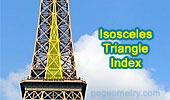# Isosceles Triangle, Theorems and Problems - Table of Content 1Some key concepts to keep in mind when solving problems involving isosceles triangles in geometry include:

1. Definition: An isosceles triangle is a triangle that has two sides of equal length and two angles of equal measure.

2. Base angles: The two angles opposite the equal sides of an isosceles triangle are called the base angles. They are always equal in measure.

3. Altitude: The altitude of an isosceles triangle is a line segment that is perpendicular to the base and intersects the opposite vertex. The altitude also bisects the base into two equal segments.

4. Isosceles triangle theorem: This theorem states that if two sides of a triangle are equal, then the angles opposite those sides are also equal.

5. In an isosceles triangle the exterior angle opposite to the base is equal in measure to twice the base angle.

6. Triangle sum theorem: This theorem states that the sum of the angles in a triangle is always 180 degrees.

When solving problems involving isosceles triangles, it can be helpful to use these concepts to identify relationships between angles and sides, and to apply the appropriate theorem or formula to find missing measures.

Geometry Problem 1525 and a Thematic Poem.
Unveiling the Secrets of an Equilateral Triangle in Right Triangle Geometry: Finding the Midpoint Distance between Segments.

Geometry Problem 1524 and a Thematic Poem.
Unlock the Mystery of Parallelograms: Discover the Length of Segment between the Intersecting Angle Bisectors.

Geometry Problem 1523 and a Thematic Poem.
Discover How to Calculate the Length of the Altitude in an Isosceles Triangle - Get Expert Geometry Tips Now! .

Geometry Problem 1513 and a Thematic Poem.
Solving the base in a Right Trapezoid with Double Angle and Sum of Two Sides. Difficulty Level: High School.

Geometry Problem 1511 and Poem.
Finding the Altitude of an Isosceles Triangle Using Distances from a Point on the Extension of the Base. Difficulty Level: High School.

Geometry Problem 1508.
Understanding Triangle Geometry: Isosceles, Equilateral, Quadrilateral, Angular. Difficulty Level: High School.

Geometry Problem 1499.
Triangle, Angles, Cevian, Congruence, Isosceles, Equilateral, Auxiliary Construction.

Geometry Problem 1497.
Triangle, Transversal, Same Ratio, Proportionality, Isosceles, Double Angle.

Geometry Problem 1496.
Triangle, Congruence, Isosceles, Double Angle, 30 Degrees.

Geometry Problem 1488.
Right Triangle, Altitude, Incircle, Tangent, Measurement.

Geometry Problem 1487.
Right Triangle, Altitude, Incircle, Tangent, Measurement.

Geometry Problem 1483.
Isosceles Right Triangle, Excenter, Perpendicular, Measurement.

Geometry Problem 1415.
Right Triangle, Altitude, Incircle, Excircle, Tangency Points, Isosceles Triangle.

Geometry Problem 1414.
Right Triangle, Altitude, Incircle, Excircle, Tangency Points, Isosceles Triangle.

Geometry Problem 1413.
Right Triangle, Incircle, Excircle, Tangency Points, Isosceles Right Triangle.

Geometry Problem 1411.
Right Triangle, Incircle, Excircle, Tangency Points, Isosceles Right Triangle.

Geometry Problem 1401.
Right Triangle with three circles on the sides, Isosceles, Diameter, Center, Tangent, Congruence.

Geometry Problem 1398.
Isosceles Triangle, Circle, Radius, Perpendicular, Measurement.

Geometry Problem 1387.
Quadrilateral, Double Angle, Congruence, Isosceles Triangle.

Geometry Problem 1386.
Thabit ibn Qurra (826 -901) Theorem and more conclusions, generalized Pythagorean Theorem to any triangle.

Geometry Problem 1377.
Isosceles Triangle, Interior Cevian, Equal Sum of Exradii, Excircle.

Geometry Problem 1376.
Isosceles Triangle, Interior Cevian, Excircles, Tangency Points, Parallel Lines.

Geometry Problem 1375.
Isosceles Triangle, Interior Cevian, Exradius, Excircle, Altitude to the Base.

Geometry Problem 1374.
Isosceles Triangle, Exterior Cevian, Incircle, Excircle, Tangency Points, Parallel Lines.

Geometry Problem 1373.

Problem 1341.
Geometry Problem 1341: Isosceles Triangle, 80-20-80 Degrees, Circumcenter, Angle Bisector.

Problem 1340.
Triangle, Incenter, Concentric Circles, Isosceles Triangles, Congruence.

Geometry Problem 1324.
Quadrilateral, Diagonal, 45 Degrees, Angle Bisector, Isosceles Triangle, Congruence.

Geometry Problem 1287.
Triangle, 40-100-40 Degrees, Congruence, Area, Metric Relations, Measurement.

Geometry Problem 1280.
Quadrilateral, Perpendicular Diagonals, Isosceles Right Triangles, 45 Degrees, Collinear Points.

Geometry Problem 1274.
Isosceles Triangle, 80-20-80 Degrees, Area, Inradius, Circumradius, Angle Bisector, Metric Relations, Measurement.

Geometry Problem 1272.
Isosceles Triangle, Median, Midpoint, Perpendicular, 90 Degrees, Angles, Congruence.

Geometry Problem 1262.
Isosceles Triangle, Altitude, Medians, 90 Degrees, Perpendicular.

Geometry Problem 1149.
Isosceles Triangle, Altitude, Cevian, Incircles, Tangent, Congruence.

Geometry Problem 1129.
Isosceles Triangle, Circumcenter, Incenter, Parallel Lines, Perpendicular Lines.

Geometry Problem 1120.
Isosceles Right Triangle, 120 Degree, Angle, Equilateral, Metric Relations.

Geometry Problem 1114.
Right Triangle, Angle Trisection, Isosceles, Congruence.

Geometry Problem 1075.
Quadrilateral, Right Triangle, 90 Degrees, Isosceles, Midpoint, Distance.

Geometry Problem 1042.
Scalene Triangle, Isosceles, Equal Angles, Parallelogram, Congruence.

Geometry Problem 1040.
Isosceles Triangle, Transversal Line, Metric Relations.

Geometry Problem 1039.
Isosceles Triangle, Circle, Tangent Line, Parallel Line.

Geometry Problem 1038.
Isosceles Triangle, Angle, 80, 20, 25, 65 Degrees.

Geometry Problem 1000.
Scalene Triangle, Isosceles, Angle, 120 Degree, Midpoint, Distance, Equilateral, Congruence, Perpendicular, Metric Relations.

Geometry Problem 998.
Scalene Triangle, Isosceles, Angle, 120 Degree, Midpoint, Equilateral, Congruence.

Geometry Problem 991.
Triangle, Incircle, Tangency Points, Isosceles, Midpoint, Collinearity, Congruence, Circle.

Geometry Problem 958.
Isosceles Triangle, Altitude, 45 Degrees, Perpendicular, Angle Bisector, Metric Relations.

Geometry Problem 886
Right Triangle, Incenter, Angle Bisector, Perpendicular, 45 Degrees, Concyclic Points, Isosceles Right Triangle. GeoGebra, HTML5 Animation for Tablets (iPad, Nexus).

Geometry Problem 874
Isosceles Triangle, Circumcircle, Circumcenter, Congruence.

Geometry Problem 867
Isosceles Triangle, Median, Perpendicular, Angle, Congruence.

Geometry Problem 859
Isosceles Right Triangle, Angles,18, 45 Degrees, Congruence, Perpendicular bisector.

Go to Page: Previous | 1 | 5 | 10 | Next